Quiz in PowerPoint using VBA

There are many ways to achieve the same task, for instance a Quiz Show can be simulated in many different ways & without using VBA. This demonstration is merely an indication of how it could be done. VBA lets you reuse the same slide for displaying the questions and accepting user input. The quiz has a time limit of 1 minute and makes use of my tmr routine to time itself, the timer routine can be dealt better in PPT2000 or later by making use of the SetTimer API. I've deliberately avoided the use of any Activex control (like a radio button to accept user choice) instead I have made use of the Unicode Character property to simulate a user choice click. To understand the code click here

To download the presentation click here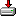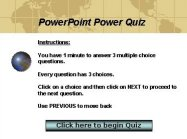The first slide displays the instructions regarding the quiz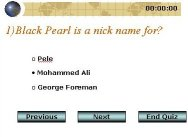The second slide will be recycled for displaying all the questions and accepting the answers from the user.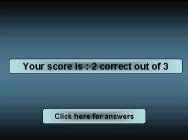Finally the third slide to list the score.

Understanding code:
The show makes use of Arrays to store information pertaining to the quiz. Qs: String array to store the questions, Choices: String array to store the multiple choices to be displayed, Ans: Integer array to store all the correct answers, UserAns: Integer array to store the choice made by the user. All of these are initialized in the BeginQuiz routine when the user clicks to attempt the quiz on the first slide.

' --------------------------------------------------------------------------------
' --------------------------------------------------------------------------------
' You are free to use this code within your own applications, add-ins,
' documents etc but you are expressly forbidden from selling or
' otherwise distributing this source code without prior consent.
' This includes both posting free demo projects made from this
' code as well as reproducing the code in text or html format.
' --------------------------------------------------------------------------------

`'General Declarations`
`' The number of questions in the quiz`
` Const NOOFQS = 3  `
`'Used to manipulated the unicode values of bulleted lists`
```Const UD_CODE_1 = 111

Const UD_CODE_2 = 8226

Public QNo As Integer

Public ExitFlag As Boolean

Public Qs() As String

Public Choices() As String

Public Ans() As Integer

Public UserAns() As Integer

```
`Sub BeginQuiz()`
`Dim Ctr As Integer`
`' Resizing the arrays declared in the general declarations of the module`
```ReDim Qs(NOOFQS)

ReDim Ans(NOOFQS)

ReDim UserAns(NOOFQS)

ReDim Choices(NOOFQS, 3)```
`' All the questions`
```Qs(0) = "1) What does Narcissistic mean?"

Qs(1) = "2) What does Confidant mean?"

Qs(2) = "3) Black Pearl is a nick name for?"

```
```' Set all user answers to -1. -1 indicates that it not answered.

For Ctr = 0 To NOOFQS - 1

UserAns(Ctr) = -1

Next Ctr```
` `
`' All the choices 3 each for a question`
```Choices(0, 0) = " Very Sleepy"

Choices(0, 1) = " Indecisive"

Choices(0, 2) = " Very Vain"

Choices(1, 0) = " Excessive Pride"

Choices(1, 1) = " Trusted Friend"

Choices(1, 2) = " Secret"

Choices(2, 0) = " Pele"

Choices(2, 1) = " Mohammed Ali"

Choices(2, 2) = " George Foreman"```
```' Provide the answer list here.

' Ans(0) = 0 means that the answer to the 1st Q is the 1st choice.

' Ans(1) = 1 means that the answer to the 2nd Q is the 2nd choice.

' Ans(2) = 1 means that the answer to the 3rd Q is the 2nd choice.

Ans(0) = 0

Ans(1) = 1

Ans(2) = 1

' Set the variable to the 1st question

QNo = 1 ```
```' Routine which sets the questions and choices to the

' text property of the shapes on the slide```
`Call AssignValues`
`' Move to the quiz slide `
```With SlideShowWindows(1)

.View.GotoSlide (.Presentation.Slides("QSlide").SlideIndex)

End With```
`'Start the timer, note that timer routine is commented out in the download file`
`Call Tmr`
`End Sub`
` `

The quiz slide is used over and over again to display the questions and accepts the answers. The routine which refreshes the questions and the multiple choices is AssignValues and the 3 routines which accept user input are ButtonChoice1, ButtonChoice2 and ButtonChoice3 (these have been assigned to the 3 textboxes which display the choices). The radio button type of simulation is achieved using the Unicode Character property of a Bulleted List which is manipulated by SetBulletUnicode. SetBulletUnicode takes two parameters: name of textbox to set the bullet and the Unicode value of the type of bullet.

`Sub AssignValues()`
```' Set all the text box Unicode character property

' to the default value simulating FALSE```
```SetBulletUnicode "Choice1", UD_CODE_1

SetBulletUnicode "Choice2", UD_CODE_1

SetBulletUnicode "Choice3", UD_CODE_1

```
```' Now based on the value stored in the UserAns array

'assign TRUE value to the appropriate text box```
```Select Case UserAns(QNo - 1)

Case 1

SetBulletUnicode "Choice1", UD_CODE_2

Case 2

SetBulletUnicode "Choice2", UD_CODE_2

Case 3

SetBulletUnicode "Choice3", UD_CODE_2

End Select```
```' Based on the current question number assign appropriate

' text values to the text boxes```
```With SlideShowWindows(1).Presentation.Slides("QSlide")

.Shapes(1).TextFrame.TextRange.Text = Qs(QNo - 1)

.Shapes("Choice1").TextFrame.TextRange.Text = Choices(QNo - 1, 0)

.Shapes("Choice2").TextFrame.TextRange.Text = Choices(QNo - 1, 1)

.Shapes("Choice3").TextFrame.TextRange.Text = Choices(QNo - 1, 2)

End With```
```End Sub

```
`Sub SetBulletUnicode(ShapeName As String, Code As Integer)`
```With SlideShowWindows(1).Presentation.Slides("QSlide") _

.Shapes(ShapeName).TextFrame.TextRange.ParagraphFormat.Bullet

.UseTextFont = msoTrue

.Character = Code

End With```
`End Sub`
```' The 3 routines below store 1,2 or 3 in the UserAns array

' to correspond to which button the user clicked```
`Sub ButtonChoice1()`
```UserAns(QNo - 1) = 1

AssignValues```
`End Sub`
`Sub ButtonChoice2()`
```UserAns(QNo - 1) = 2

AssignValues```
`End Sub`
```

Sub ButtonChoice3()```
```UserAns(QNo - 1) = 3

AssignValues```
`End Sub`

Naviagted thru with the help of the Next and Previous buttons which have macros NextSlide and PreviousSlide assigned to them respectively.

`Sub NextSlide()`
```' Check the current question no. If it is less than the

' total number of questions then display the next question

' else goto the score slide ```
```If QNo < NOOFQS Then

QNo = QNo + 1

SlideShowWindows(1).Presentation.Slides("QSlide").Shapes(1) _

.TextFrame.TextRange.Text = Qs(QNo - 1)

AssignValues

Else

Call StopQuiz

End If```
`'Let the screen redraw all the elements completely`
`DoEvents`
```End Sub

Sub PreviousSlide()```
```Static X As Integer

If QNo > 1 Then

QNo = QNo - 1

AssignValues

End If```
`End Sub`

Finally the StopQuiz routine is invoked to move to the end of quiz. The StopQuiz takes an optional parameter EndType. This is used to ascertain whether the user answered all the questions or did he simply press the End Quiz button.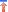```
Sub StopQuiz(Optional EndType As Boolean = False)```
```
' EndType is used as a boolean Flag to indicate whether

' the user ran out of time or whether it was normal exit```
```Dim ScoreCard As Integer

Dim Ctr As Integer

ExitFlag = True

With SlideShowWindows(1)```
`' Compare the correct answers with those answered by the user   `
```    For Ctr = 0 To NOOFQS - 1

If Ans(Ctr) = UserAns(Ctr) Then ScoreCard = ScoreCard + 1

Next Ctr```
`' Check whether user pressed End Quiz`
```  If EndType = False Then

.Presentation.Slides("EndSlide").Shapes("Closing") _

.TextFrame.TextRange.Text = "Your score is : " _

& ScoreCard & " correct out of " & NOOFQS

Else

.Presentation.Slides("EndSlide").Shapes("Closing") _

.TextFrame.TextRange.Text = "Sorry!!! Either you " & _

"ran out of time or you chickened out" _

& vbCrLf & "Better luck next time." & vbCrLf _

& "Your score is: " & ScoreCard & _

" correct out of " & NOOFQS

End If

.View.GotoSlide (.Presentation.Slides("EndSlide").SlideIndex)

End With```
`End Sub`